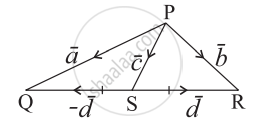# In the given figure express cc¯ and dd¯ in terms of aa¯ and bb¯. - Mathematics and Statistics

Sum

In the given figure express bar"c" and bar"d" in terms of bar"a" and bar"b".#### Solution

bar"PQ" = bar"PS" + bar"SQ"

∴ bar"a" = bar"c" - bar"d"   ...(1)

bar"PR" = bar"PS" + bar"SR"

∴ bar"b" = bar"c" + bar"d"    ....(2)

Adding equations (1) and (2), we get

bar"a" + bar"b" = (bar"c" - bar"d") + (bar"c" + bar"d") = 2bar"c"

∴ bar"c" = 1/2 (bar"a" + bar"b") = 1/2 (bar"a" + bar"b")

= (2bar"b" - (bar"a" + bar"b"))/2

= 1/2(bar"b" - bar"a") = 1/2bar"b" - 1/2bar"a"

Hence, bar"c" = 1/2 bar"a" + 1/2bar"b" and bar"d" = 1/2bar"b" - 1/2bar"a"

Concept: Vectors and Their Types
Is there an error in this question or solution?